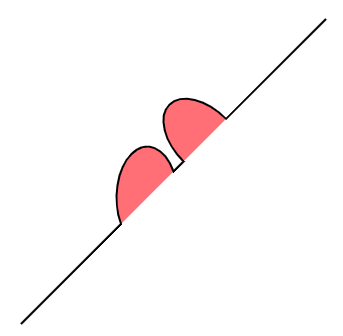## 【SVG】SVG的夺命利器——path

#### path元素的能力

`path`元素是SVG基本形状中最强大的一个，它不仅能创建其他基本形状，还能创建更多其他形状。

path元素的形状是通过属性d来定义的，d属性通过“命令和坐标”的序列来控制整个path绘制的路径

#### path的坐标命令

• M = moveto
• L = lineto
• H = horizontal lineto
• V = vertical lineto
• C = curveto
• S = smooth curveto
• Q = quadratic Bézier curve
• T = smooth quadratic Bézier curveto
• A = elliptical Arc
• Z = closepath

##### 直线命令

• M（moveto）：需要两个参数（x轴和y轴坐标，移动到的点的x轴和y轴的坐标
• L（lineto）：需要两个参数（x轴和y轴坐标），它会在当前位置和最新的位置（L前面画笔所在的点）之间画一条线段。
• H（horizontal lineto）：一个参数，标明在x轴移动到的位置，绘制水平线
• V（vertical lineto）：一个参数，标明在y轴移动到的位置，绘制垂直线
• Z（ closepath）：从当前点画一条直线到路径的起点

``````<svg width="100px" height="100px" version="1.1" xmlns="http://www.w3.org/2000/svg">
<path d="M10 10 H 90 V 90 H 10 L 10 10"/>
</svg>
``````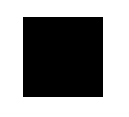``````<svg width="100px" height="100px" version="1.1" xmlns="http://www.w3.org/2000/svg">
<!-- 利用Z命令 -->
<path d="M10 10 H 90 V 90 H 10 Z"/>
<!-- 利用V命令 -->
<path d="M10 10 H 90 V 90 H 10 V 10"/>
</svg>
``````

``````<svg width="100px" height="100px" version="1.1" xmlns="http://www.w3.org/2000/svg">
<path d="M10 10 h 80 v 80 h -80 Z" />
</svg>
``````
##### 曲线命令

C（curveto）三次贝塞尔曲线

(x,y)表示的是曲线的终点，(x1,y1)是起点的控制点，(x2,y2)是终点的控制点。控制点描述的是曲线起始点的斜率，曲线上各个点的斜率，是从起点斜率到终点斜率的渐变过程。

``````C x1 y1, x2 y2, x y
c dx1 dy1, dx2 dy2, dx dy
``````

``````<svg width="200px" height="200px" version="1.1" xmlns="http://www.w3.org/2000/svg">
<path d="M10 10 C 20 20, 40 20, 50 10" stroke="black" fill="transparent"/>
</svg>
``````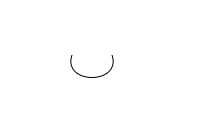``````<svg width="200px" height="200px" version="1.1" xmlns="http://www.w3.org/2000/svg">
<path d="M130 110 C 120 140, 180 140, 170 110" stroke="black" fill="transparent"/>
<circle cx="130" cy="110" r="2" fill="red"/>
<circle cx="120" cy="140" r="2" fill="red"/>
<line x1="130" y1="110" x2="120" y2="140" style="stroke:rgb(255,0,0);stroke-width:2"/>
<circle cx="180" cy="140" r="2" fill="red"/>
<circle cx="170" cy="110" r="2" fill="red"/>
<line x1="180" y1="140" x2="170" y2="110" style="stroke:rgb(255,0,0);stroke-width:2"/>
</svg>
``````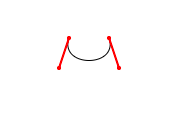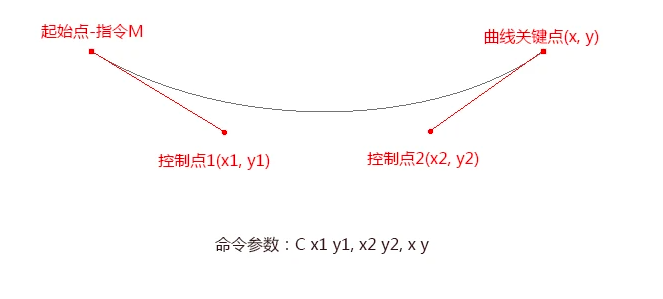S（smooth curveto）简写的三次贝塞尔曲线

S其实是创建一个特殊的三次贝塞尔曲线。它特殊的地方就是当一个点某一侧的控制点是它另一侧的控制点的对称也就是保持斜率不变。

(x,y)表示的是曲线的终点，(x2,y2)是既是终点的控制点也是起点的控制点。

``````S x2 y2, x y
s dx2 dy2, dx dy
``````

``````<svg width="400px" height="200px" version="1.1" xmlns="http://www.w3.org/2000/svg">
<path d="M 10 50 C 40 20, 120 20, 150 50" stroke="black" fill="transparent"/>
</svg>
``````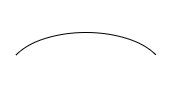``````<svg width="400px" height="200px" version="1.1" xmlns="http://www.w3.org/2000/svg">
<path d="M 10 50 C 40 20, 120 20, 150 50 S 260 80, 290 50" stroke="black" fill="transparent"/>
</svg>
``````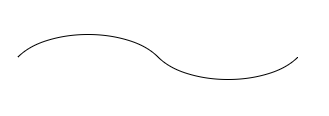``````<svg width="400px" height="200px" version="1.1" xmlns="http://www.w3.org/2000/svg">
<path d="M 10 50 C 40 20, 120 20, 150 50 S 260 80, 290 50" stroke="black" fill="transparent"/>
<circle cx="10" cy="50" r="2" fill="red"/>
<circle cx="40" cy="20" r="2" fill="red"/>
<line x1="10" y1="50" x2="40" y2="20" style="stroke:rgb(255,0,0);stroke-width:1"/>

<circle cx="120" cy="20" r="2" fill="red"/>
<circle cx="150" cy="50" r="2" fill="red"/>
<line x1="120" y1="20" x2="150" y2="50" style="stroke:rgb(255,0,0);stroke-width:1"/>

<circle cx="180" cy="80" r="2" fill="blue"/>
<circle cx="180" cy="80" r="2" fill="red"/>
<circle cx="260" cy="80" r="2" fill="red"/>
<line x1="150" y1="50" x2="180" y2="80" style="stroke:blue;stroke-width:1"/>
<line x1="290" y1="50" x2="260" y2="80" style="stroke:rgb(255,0,0);stroke-width:1"/>
</svg>
``````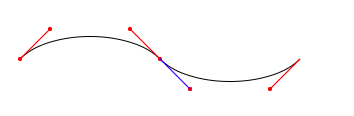``````Q x1 y1, x y
q dx1 dy1, dx dy
``````

``````<svg width="200px" height="200px" version="1.1" xmlns="http://www.w3.org/2000/svg">
<path d="M 10 80 Q 95 20 180 80" stroke="black" fill="transparent"/>
</svg>
``````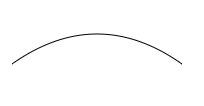``````<svg width="200px" height="200px" version="1.1" xmlns="http://www.w3.org/2000/svg">
<path d="M 10 80 Q 95 20 180 80" stroke="black" fill="transparent"/>
<circle cx="10" cy="80" r="2" fill="red"/>
<circle cx="95" cy="20" r="2" fill="red"/>
<circle cx="180" cy="80" r="2" fill="red"/>
<line x1="10" y1="80" x2="95" y2="20" style="stroke:rgb(255,0,0);stroke-width:1"/>
<line x1="95" y1="20" x2="180" y2="80" style="stroke:rgb(255,0,0);stroke-width:1"/>
</svg>
``````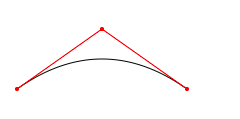``````T x y
t dx dy
``````

``````<svg width="400px" height="200px" version="1.1" xmlns="http://www.w3.org/2000/svg">
<path d="M10 80 Q 50 10, 90 80 T 170 80" stroke="black" fill="transparent"/>
</svg>
``````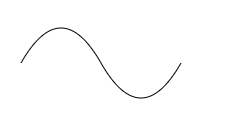``````<svg width="400px" height="200px" version="1.1" xmlns="http://www.w3.org/2000/svg">
<path d="M10 80 Q 50 10, 90 80 T 170 80" stroke="black" fill="transparent"/>

<circle cx="10" cy="80" r="2" fill="red"/>
<circle cx="50" cy="10" r="2" fill="red"/>
<line x1="10" y1="80" x2="50" y2="10" style="stroke:rgb(255,0,0);stroke-width:1"/>

<circle cx="90" cy="80" r="2" fill="red"/>
<line x1="90" y1="80" x2="50" y2="10" style="stroke:rgb(255,0,0);stroke-width:1"/>

<circle cx="170" cy="80" r="2" fill="blue"/>
<circle cx="130" cy="150" r="2" fill="blue"/>
<line x1="90" y1="80" x2="130" y2="150" style="stroke:rgb(0,0,255);stroke-width:1"/>
<line x1="130" y1="150" x2="170" y2="80" style="stroke:rgb(0,0,255);stroke-width:1"/>
</svg>
``````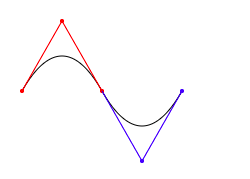A（elliptical Arc）弧形

A命令用于画弧形。

``````A rx ry x-axis-rotation large-arc-flag sweep-flag x y
a rx ry x-axis-rotation large-arc-flag sweep-flag dx dy
``````

• 弧形命令A前两个参数rx和ry分别是x轴半径和y轴半径。
• 弧形命令A的第三个参数表示弧形的旋转情况。
• large-arc-flag决定弧线是大于还是小于180度，0表示小角度弧，1表示大角度弧。
• sweep-flag表示弧线的方向，0表示从起点到终点沿逆时针画弧，1表示从起点到终点沿顺时针画弧。
• x：结束点x坐标。
• y：结束点y坐标。

``````<svg width="400px" height="320px" version="1.1" xmlns="http://www.w3.org/2000/svg">
<path d="M10 315
L 110 215
A 30 50 0 0 1 162.55 162.45
L 172.55 152.45
A 30 50 -45 0 1 215.1 109.9
L 315 10" stroke="black" fill="red" stroke-width="2" fill-opacity="0.5"/>
</svg>
``````